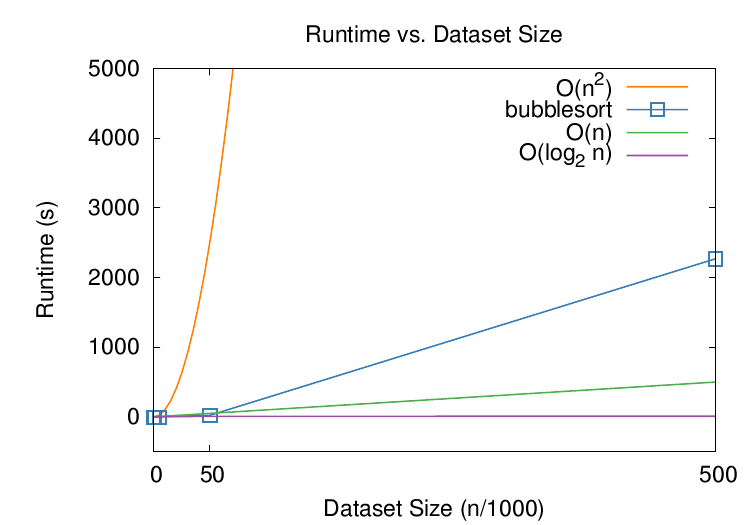# Lab 2: Timer Class

## Objectives

To construct a simple C++ timing object.

## Assignment

1. The central purpose of clever data structures is to improve code execution time and storage requirements. To detect such improvements, we will need a method to measure execution time. In this lab, you are to construct a `timer_t` class and use it to measure the execution time of the sorting algorithm, bubblesort.
2. The `timer_t` class should have design prototype: ```class timer_t { public: // constructors timer_t(); // member functions void start(void); void stop(void); double elapsed_us(); double elapsed_ms(); double elapsed_s(); private: double ts; double te; double tt; }; ```
3. The `start()` and `stop()` methods simply capture timestamps and `eslapsed_s()` then calculates and returns the elapsed time between the two timestamps in secionds (the other two return the time elapsed in milliseconds and microseconds).
4. Use the buil-tin system call `gettimeofday()` to obtain a timestamp of the current time, in seconds and microseconds since "the epoch" (January 1, 1970).
5. Take care to: ```#include <sys/time.h> ```
6. Use the `timer_t` class to measure the execution time of the sorting routine, bubblesort, ```void bubblesort(int A[]) { for(int i=1; i<SIZE; i++) for(int j=SIZE-1; j>=i; j--) if(A[j-1]>A[j]) swap(A[j-1],A[j]); } ```
7. Use your `array_t` class to store random integer values, e.g., ```#include "array.h" array_t<int> A(atoi(argv)); A.randseed(); A.randfill(); ```
8. You will need to write a public member function `bubblesort()` for the `array_t` class:
1. redefine the above sort function so that it operates on the array stored by `array_t`
2. replace `SIZE` with the array's size
9. Your `main()` routine should then include: ```int main() { // read in desired array size // declare array variable A ... // start the timer A.bubblesort(); // stop the timer // report the timer value } ```
10. Print the sorted values for a small list to make sure the sort is working. Then measure and report (turn in) the time required to sort 5, 50, 500, 5,000, 50,000, and 500,000 integers. Make sure everything is working before running with 500K integers—it takes a while! (On my Mac it took about 40 min.)
11. What we want in this lab are two things:
1. a `bubblesort.dat` data file that contains two columns of numbers: the first column is n, the size of the array, and the second column is t, the time taken to sort the array.
2. a plot of the `bubblesort.dat` data file showing the run time of bubblesort graphically against various other theoretical bounds, e.g., O(n), O(log n), O(n2).
12. Use `gnuplot` to plot the resultant graph. Here is a gnuplot script (call it `plot.plt`) that plots something reasonable: ```reset # this should work but need to add fontpath -- could be system-specific set fontpath \ "/sw/share/texmf-dist/fonts/type1!" \ "/usr/share/texmf/fonts/type1!" \ "/usr/local/teTeX/share/texmf-dist/fonts/type1!" \ "/usr/share/texmf-texlive/fonts/type1!" set term postscript eps enhanced "NimbusSanL-Regu" 22 \ fontfile "uhvr8a.pfb" fontfile "usyr.pfb" fontfile "utmri8a.pfb" set xrange [*:*] set xtics (0,50,500) set yrange [-500:5000] set key top right set title "Runtime vs. Dataset Size" set ylabel "Runtime (s)" set xlabel "Dataset Size (n/1000)" set output 'plot.eps' plot \ x**2 title "O(n^2)" \ with lines lt 1 lw 3 lc rgb "#ff7f00", \ 'bubblesort.dat' using (\$1)/1000:(\$2)/1000 title "bubblesort" \ with linespoints pt 4 ps 2 lt 1 lw 3 lc rgb "#377eb8",\ x title "O(n)" \ with lines lt 1 lw 3 lc rgb "#4daf4a",\ log10(x)/log10(2) title "O(log_2 n)" \ with lines lt 1 lw 3 lc rgb "#984ea3" ``` Run the above script with the command ```gnuplot plot.plt ```
13. The above gnuplot file will create an encapsulated PostScript file, or `.eps` file; convert this to something more useful, like a PDF plot, via the command ```epstopdf --nocompress -o=plot.pdf plot.eps ```
14. In fact, while you're at it, stick both of the above `gnuplot` and `epstopdf` commands into a `plot:` target in your `Makefile`. Then, rewrite your program to accept the size of array you want as input argument `argv` and have it write out the time to sort to `std::cout`. This way, you can script the run (and plot) within the `Makefile` so that a `run` target executes the following: ``` ./main 5 >> bubblesort.dat ./main 500 >> bubblesort.dat ./main 5000 >> bubblesort.dat ./main 50000 >> bubblesort.dat ./main 500000 >> bubblesort.dat ``` then all you'll need to do to "automate" the entire process is type: ```make main make run make plot ```
15. The end result of this lab is a plot like you see below. You'll notice that bubblesort didn't fare quite as badly as its theoretical prediction of O(n2) but you can see that it is taking on a similar shape. If you were to run the program on n=5,000,000, it would probably take over an hour to execute.## Turn in

Turn in all of your code, in one `tar.gz` archive of your lab02/ directory, including:
1. A `README` file containing
1. Course id--section no
2. Name
3. Lab description
4. Brief solution description (e.g., program design, description of algorithm, etc., however appropriate).
5. Lessons learned, identified interesting features of your program
6. Any special usage instructions
2. `Makefile`
3. source code (`.h` headers and `.cpp` source)
4. object code (do a `make clean` before `tar`)

## How to hand in

See `sendlab` notes